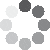Enter parameters LENGTH, WIDTH & DEPTH
Enter parameters
LENGTH, WIDTH & DEPTH
Length:
ft
• inches (in)
• feet (ft)
• yards (yd)
• centimeters (cm)
• meters (m)
Width:
ft
• inches (in)
• feet (ft)
• yards (yd)
• centimeters (cm)
• meters (m)
Depth:
in
• inches (in)
• feet (ft)
• yards (yd)
• centimeters (cm)
• meters (m)
Density:
105 - Gravel lb/ft³
• Gravel - 105 lb/ft³
• Custom
Price per unit of mass:
t
• pound (lb)
• US short ton (t)
• imperial-long ton (long t)
• kilogram (kg)
Price per unit of volume:
yd³
• cubic yards (yd³)
• cubic feet (ft³)
• cubic inches (in³)
• cubic centimeters (cm³)
• cubic meters ()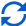Result
Area:
0
ft²
• square feet (ft²)
• square inches (in²)
• square yards (yd²)
• square miles (mi²)
• acres (ac)
• square centimeters (cm²)
• square meters ()
Volume:
0
yd³
• cubic yards (yd³)
• cubic feet (ft³)
• cubic inches (in³)
• cubic centimeters (cm³)
• cubic meters ()
Weight:
0
t
• pounds (lb)
• US short tons(t)
• imperial-long tons (long t)
• kilograms (kg)
Cost:
$Enter parameters AREA & DEPTH Area: ft² • square feet (ft²) • square inches (in²) • square yards (yd²) • square miles (mi²) • acres (ac) • square centimeters (cm²) • square meters () Depth: in • inches (in) • feet (ft) • yards (yd) • centimeters (cm) • meters (m) Density: 105 - Gravel lb/ft³ • Gravel - 105 lb/ft³ • Custom Price per unit of mass: t • pounds (lb) • US short tons (t) • imperial-long tons (long t) • kilograms (kg) Price per unit of volume: yd³ • cubic yards (yd³) • cubic feet (ft³) • cubic inches (in³) • cubic centimeters (cm³) • cubic meters ()Result Volume: 0 yd³ • cubic yards (yd³) • cubic feet (ft³) • cubic inches (in³) • cubic centimeters (cm³) • cubic meters () Weight: 0 t • pounds (lb) • US short tons (t) • imperial-long tons (long t) • kilograms (kg) Cost:$
Enter parameters
VOLUME
Volume:
yd³
• cubic yards (yd³)
• cubic feet (ft³)
• cubic inches (in³)
• cubic centimeters (cm³)
• cubic meters ()
Density:
105 - Gravel lb/ft³
• Gravel - 105 lb/ft³
• Custom
Price per unit of mass:
t
• pounds (lb)
• US short tons (t)
• imperial-long tons (long t)
• kilograms (kg)
Price per unit of volume:
yd³
• cubic yards (yd³)
• cubic feet (ft³)
• cubic inches (in³)
• cubic centimeters (cm³)
• cubic meters ()Result
Weight:
0
t
• pounds (lb)
• US short tons (t)
• imperial-long tons (long t)
• kilograms (kg)
Cost:
$(1 votes, average: 5.00 out of 5)Loading... Similar Calculators: Real-time graphics. Make the calculations and see the changes. Real-time graphics. Make the calculations and see the changes. Real-time graphics. Make the calculations and see the changes. Embed Similar Calculators:Contents: ## How to calculate how much gravel do you need? We know that estimating the amount of gravel you will require when redesigning your driveway can be difficult. You want to be as accurate as possible without over or underestimating the amount of gravel you will need. That’s why we built our online gravel calculator. Keep reading to see just how easy it is to use, what it calculates and how it does it. Our gravel calculator will make you more efficient and can even save you some money! But first… ## How does our gravel calculator work?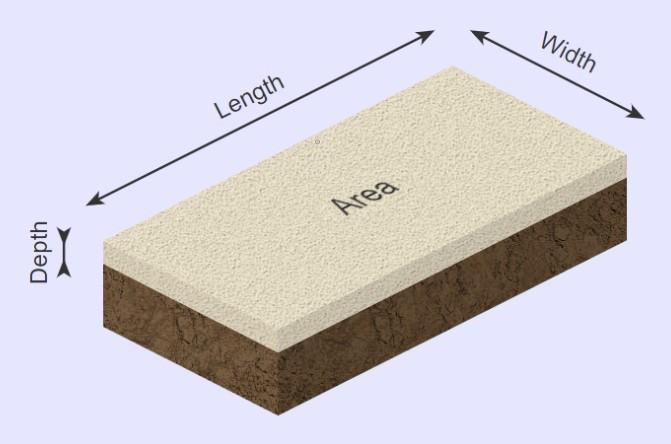Our calculator first works out the area and volume of the driveway base from the measurements you provide. In addition, it can calculate the total weight of the material you will need using the formulae detailed below: $$Area = Length × Width$$ $$Volume = Area × Depth$$ $$Weight\,of\,Gravel = Density\,of\,Gravel × Volume$$ Want to know the best part? If you know the price per unit mass or price per unit volume, the calculator can then calculate the total cost of the gravel using the formulae: $$Cost = Price\,per\,Unit\,Mass × Weight$$ or $$Cost = Price\,per\,Unit\,Volume × Volume$$ In addition, You can calculate the price per unit mass/volume for the gravel you have purchased using the formulae: $$Price\,Per\,Unit\,Mass = {Total\,Cost\,of\,Gravel \over Weight\,of\,Gravel}$$ or $$Price\,Per\,Unit\,Volume = {Total\,Cost\,of\,Gravel \over Volume\,of\,Gravel}$$ Confused? See below for three full examples starting with different initial criteria. Otherwise, enter your measurements and values for your driveway in our online gravel calculator! ## Examples of calculations 1. Gravel part of a driveway with standard 105 lb/gravel. Let’s say that I want to cover an area of my driveway measuring 3 feet by 2 feet. I want to ensure equal gravel coverage to a depth of 2 inches. I am using normal gravel (105 lb/ which costs$50 per US short ton.

I want to estimate the amount of space (volume) the gravel will occupy, how much it will weigh and how much the material will cost.

Now, I would enter these measurements into the calculator.

The gravel estimator would then perform the following calculations:

$$Driveway\,Area = Length × Width = 3 ft × 2 ft = 6 ft^2$$

$$Driveway\,Volume = Area × Depth = 6 ft^2 × 2in=1 ft^3$$

$$Weight = Density × Volume =105\,lb/ft^3 × 1ft^3 =105\,lb$$

$$Cost = Weight × Price\,Per\,Unit\,Mass = 105\,lb × 50\,/t= 2.63$$

What if I’m using a different aggregate, and I don’t know its density? 🤔

Well, our calculator allows you to enter any custom density value, which you can calculate using the following formula:

$$Density = {Weight \over Volume}$$

The second example will show you how to use this.

2. Cover 4 square feet of driveway with custom aggregate.

Let’s say that I have a part of a driveway to fill that measures 4  (for example, a square measuring 2 ft x 2 ft) and I want to ensure equal coverage to a depth of 3 inches.

I will use a custom aggregate. I am not sure of its density, but it weighs 160 lb per two cubic feet and costs $45 for each US short ton. First, in order to calculate the density of the aggregate I would do the following calculation: $$Density = {Weight \over Volume} = {160\,lb \over 2 ft^3} = 80\,lb/ft^3$$ I would then enter the measurements to calculate the volume, weight and cost of the aggregate needed. The estimator would perform the following operations: $$Volume = Area × Depth = 4ft^2 × 3\,in = 0.037\,yd^3$$ $$Weight = Density × Volume = 80\,lb/ft^3 × 0.037\,yd^3 = 0.04\,t$$ $$Cost = Weight × Price\,Per\,Unit\,Mass = 0.04\,t × 45\,/t = 1.8$$ You might also be asking, what if I don’t know the price per unit mass or volume? Well, in this final example we will show you how to calculate it. 3. Cover 4 cubic feet of driveway calculating price per unit volume. For our final example, let’s imagine we have a volume of 4 cubic feet we need to fill with gravel (105lb/). I don’t know the price per unit volume but I can purchase 12 cubic yards for a cost of$144.

I can therefore calculate the price per unit volume using the formula:

$$Price\,Per\,Unit\,Volume = {Total\,Cost\,of\,Gravel \over Volume\,of\,Gravel} = {144 \over 12\,yd^3} = 12\,/yd^3$$

I therefore enter all these parameters into the gravel calculator, that does the following operations:

$$Weight = Density × Volume = 105\,lb/ft^3 × 4ft^3 = 420 \,lb$$

$$Cost = Volume × Price\,per\,Unit\,Volume = 420 \,lb × 12\,/yd^3 = 1.78$$

At this point, it’s likely that you are asking the following question:

## What if my measurements are in different units?

If you look back through our examples above, you’ll notice that we change between tonnes, cubic feet, cubic yards, pounds and so on (e.g. density is in pounds per cubic feet and volume is given in cubic yards of gravel).

Our calculator is able to do the conversions for you, meaning you don’t have to!

There are multiple options for the units of each measurement that are available to use.

You can enter measurements of length in inches (in), feet (ft), yards (yd), centimeters (cm) or meters (m).

Simply select the correct unit in the drop down area and our calculator will do the rest using the following conversions:

$$1\,foot= 12\,inches = 0.33\,yards = 30.48\,centimeters = 0.348\,meters$$

$$1\,US\,short\,ton = 2000\,pounds = 0.893\,imperial\,long\,ton= 907\,kg$$

Finally, what if you have multiple areas you want to gravel?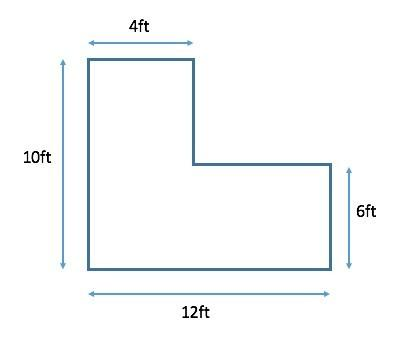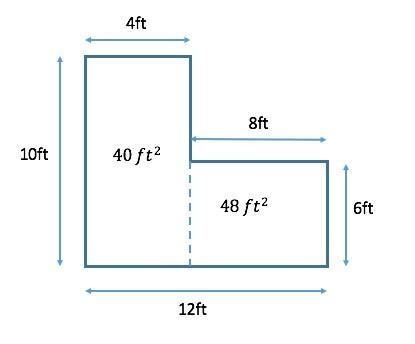Divide your driveway into rectangles, like in the example shown above. You may need to measure some additional lengths.

Work out the area of the separate shapes and then add (or subtract) these areas to give you the final area you need.

In the example above, the rectangles measure 4 ft × 10 ft= 40 ft² and 6 ft × 8 ft= 48 ft². Therefore, the total area is:

$$40 ft^2 + 48 ft^2 = 88 ft^2$$

Our gravel calculator is a powerful online tool that you can use to landscape your driveway.

Coupled with a little preparation, it will ensure that your next project will be a walk in the park! 💪

Embed this calculator on your site!Add live graphics
Copied to clipboard! Preview